## Programming-for-Output-Problems

 Question 1

Consider the following C program:

```        #include <stdio.h>
int main ()  {
int arr [] = {1,2,3,4,5,6,7,8,9,0,1,2,5}, *ip = arr+4;
printf ("%d\n", ip);
return 0;
}
```

The number that will be displayed on execution of the program is _____.

 A 5 B 6 C 7 D 8
Programming-for-Output-Problems       Arrays       GATE 2019       Video-Explanation
Question 1 Explanation: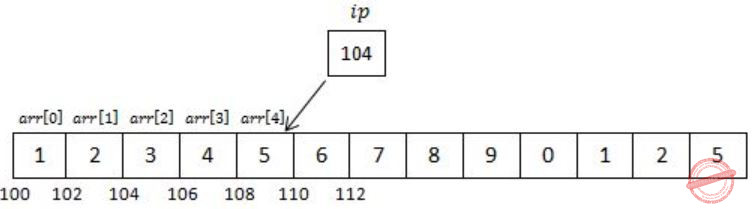We know that arr is a pointer to arr[ ] & hence arr+4 is pointer to 4th index of array (starting from 0 to 4).
Now *ip is a pointer of int type pointing to memory location 108, which is part of arr.
Hence, when we will print ip it will be equivalent to *(ip+1).
Address of ip will be incremented by 1 & value inside 110 will be printed.
 Question 2

Consider the following C program:

```         #include <stdio.h>
int jumble (int x, int y)  {
x = 2 * x + y ;
return x ;
}
int main ( )  {
int x=2, y=5 ;
y = jumble (y, x) ;
x = jumble (y, x) ;
printf ("%d \n", x) ;
return 0 ;
}
```

The value printed by the program is ______.

 A 26 B 67 C 25 D 13
Programming-for-Output-Problems       Functions       GATE 2019       Video-Explanation
Question 2 Explanation:
////////////////////////////////////Program
#include
int jumble(int x, int y)
{
printf("Inside jumble : 2*%d + %d\n", x,y);
x = 2*x +y;
return x;
}
int main()
{
int x=2, y=5;
printf("Initial x=%d, y=%d\n",x,y);
printf("1st jumble call : jumble(%d,%d)\n",y,x);
y = jumble(y,x);
printf("Value of y after 1st jumble = %d\n", y);
printf("2nd jumble call: jumble(%d,%d)\n", y,x);
x = jumble(y,x);
printf("Value of x after 2nd jumble = %d\n", x);
printf("Final : %d\n", x);
return 0;
}
////////////////////////////////////OUTPUT
Initial x=2, y=5
1st jumble call: jumble(5,2)
Inside jumble : 2*5 + 2
Value of y after 1st jumble = 12
2nd jumble call: jumble(12,2)
Inside jumble : 2*12 + 2
Value of x after 2nd jumble = 26
Final : 26
 Question 3

Consider the following C program:

```       #include <stdio.h>
int main()
{
int a[] = {2, 4, 6, 8, 10} ;
int i, sum = 0, *b = a + 4 ;
for (i = 0; i < 5; i++)
sum = sum + (*b - i) - *(b - i) ;
printf ("%d\n", sum) ;
return 0 ;
}
```

The output of the above C program is _____.

 A 3 B 7 C 11 D 10
Programming-for-Output-Problems       Control-Statement       GATE 2019       Video-Explanation
Question 3 Explanation:
///////////////////////////////////PROGRAM
#include
int main()
{
int a[] = {2,4,6,8,10};
int i, sum = 0, *b = a+4;
for(i=0; i<5; i++)
{ printf("*b, (*b-i): %d , %d\n",*b, (*b-i) );
printf("*(b-i): %d\n",*(b-i) );
printf("sum = %d + %d - %d\n",sum, (*b-i),*(b-i));
sum = sum + (*b-i) - *(b-i);
printf("sum = %d\n", sum);
}
printf("%d\n", sum);
return 0;
}
//////////////////////////////OUTPUT
*b, (*b-i): 10 , 10
*(b-i): 10
sum = 0 + 10 - 10
sum = 0
*b, (*b-i): 10 , 9
*(b-i): 8
sum = 0 + 9 - 8
sum = 1
*b, (*b-i): 10 , 8
*(b-i): 6
sum = 1 + 8 - 6
sum = 3
*b, (*b-i): 10 , 7
*(b-i): 4
sum = 3 + 7 - 4
sum = 6
*b, (*b-i): 10 , 6
*(b-i): 2
sum = 6 + 6 - 2
sum = 10
10
 Question 4

Consider the following C program:

```             #include <stdio.h>
int r()  {
static int num=7 ;
return num-- ;
}
int main ()  {
for (r(); r (); r())
printf ("%d", r());
return 0 ;
}
```

Which one of the following values will be displayed on execution of the programs?

 A 41 B 63 C 52 D 630
Programming-for-Output-Problems       Control-Statement       GATE 2019       Video-Explanation
Question 4 Explanation:
///////////////////////////PROGRAM
#include
int r()
{
int x;
static int num=7;
x =num--;
printf("num--: %d\n",x);
return x;
}
int main()
{
for(r(); r(); r())
{
printf("%d\n", r());
}
return 0;
}
//////////////////////////////OUTPUT
num--: 7
num--: 6
num--: 5
5
num--: 4
num--: 3
num--: 2
2
num--: 1
num--: 0
 Question 5

Consider the following C function.

```               void convert (int n) {
if (n < 0)
printf ("%d", n);
else {
convert (n/2);
printf ("%d", n%2);
}
}
```

Which one of the following will happen when the function convert is called with any positive integer n as argument?

 A It will print the binary representation of n in the reverse order and terminate. B It will not print anything and will not terminate. C It will print the binary representation of n and terminate. D It will print the binary representation of n but will not terminate.
Programming-for-Output-Problems       Functions       GATE 2019       Video-Explanation
Question 5 Explanation:
////////////////OUTPUT
Sequence of function calls
Convert(6)
Convert(3)
Convert(1)
Convert(0)
:
Convert(0)
:
:
It will not terminate and never produce any output.
Note:
There is no instruction which stops the loop.
 Question 6

Consider the following C program:

```       #include <stdio.h>
int main ()  {
float sum = 0.0, j = 1.0, i = 2.0;
while (i/j > 0.0625) {
j = j + j;
sum = sum + i/j;
printf ("%f \n", sum);
}
return 0;
}
```

The number of times the variable sum will be printed, when the above program is executed, is ______.

 A 5 B 2 C 7 D 10
Programming-for-Output-Problems       Control-Statement       GATE 2019       Video-Explanation
Question 6 Explanation:
///////////////////////////////// PROGRAM
#include
int main()
{
float sum= 0.0, j=1.0, i=2.0;
while(i/j > 0.0625)
{
j = j+j;
sum = sum+i/j;
printf("%f\n",sum);
}
return 0;
}
//////////////////////////////////OUTPUT
1.000000
1.500000
1.750000
1.875000
1.937500
 Question 7
Write the output of the following C program
#include <stdio.h>
int main (void)
{
int shifty;
shifty = 0570;
shifty = shifty >>4;
shifty = shifty <<6;
printf("the value of shifty is %o",shifty);
}
 A the value of shifty is 1500 B the value of shifty is 4300 C the value of shifty is 5700 D the value of shifty is 2700
Programming-for-Output-Problems       Operator       ISRO CS 2014       Video-Explanation
Question 7 Explanation:
Given, shifty = 0570; // number starts with 0 means that number is octal number.
(0570)8 = (000 101 111 000)2 (Converting octal number into binary number)
shifty = shifty >>4 ( >> right shift operator where we need to shift the 4 bits towards right side means discard last four bits )
After right shifting 4 bits the shifty consists of the following digits
shifty = (000 000 010 111) 2
shifty = shifty <<6( << left shift operator where we need to shift the 6 bits towards left side means add si bits to the end of the binary number. So the binary number becomes as follows shifty = (010 111 000 000) 2
= (2700) 8
 Question 8
The following three ‘C’ language statements is equivalent to which single statement?
y=y+1;
z=x+y;
x=x+1
 A z = x + y + 2; B z = (x++) + (++y); C z = (x++) + (y++); D z = (x++) + (++y) + 1;
Programming-for-Output-Problems       Operator       ISRO CS 2014       Video-Explanation
Question 8 Explanation:
From the below statements
y=y+1;
z=x+y;
x=x+1
First statement: “y” value is incremented by 1
Second statement : that incremented value is added to x and stored into “z”
Third statement : “y” value is incremented by 1
z = (x++) + (++y);
X++ post-increment , so it will perform action which is addition and later the value of “x” is incremented .
++y pre-increment , here first value “y” is incremented and updated value is added to value x.
Finally the result will store into z.
The sequence of operations you can find from the below statements
z=x+y; //z = x + (++y)
x=x+1 //z = (x++) + (++y)
 Question 9
How many lines of output does the following C code produce?
#include<stdio.h>
float i=2.0;
float j=1.0;
float sum = 0.0;
main()
{
while (i/j > 0.001)
{
j+=j;
sum=sum+(i/j);
printf("%f\n", sum);
}
}
 A 8 B 9 C 10 D 11
Programming-for-Output-Problems       Control Flow       ISRO CS 2014       Video-Explanation
Question 9 Explanation:
Iteration-1:
while (1.000000 > 0.001)
{
j=2.0
sum=0+1.000000;
printf("%f\n",sum); /* It will print 1.000000 */
}
Iteration-2: 1.500000
Iteration-3: 1.750000
Iteration-4: 1.875000
Iteration-5: 1.937500
Iteration-6: 1.968750
Iteration-7: 1.984375
Iteration-8: 1.992188
Iteration-9: 1.996094
Iteration-10: 1.998047
Iteration-11: 1.999023
The program will terminate after 11th iteration. So, it print 11 lines.
 Question 10
Consider the following C code.
#include
#include
void main()
{
double pi = 3.1415926535;
int a = 1;
int i;
for(i=0; i < 3; i++)
if(a = cos(pi * i/2) )
printf("%d ",1);
else printf("%d ", 0);
}
What would the program print?
 A 000 B 010 C 101 D 111
Programming-for-Output-Problems       Programming       ISRO CS 2013       Video-Explanation
Question 10 Explanation:
→The for loop will execute three times for a given i values 0,1,2.
→For a given i = 0:
a = cos(pi * 0/2) [ PI*0 is 0]
a = cos(0) = 1, if condition true and it will print 1
→For a given i = 1
a = cos (pi/2) [pi*1 is pi]
a=cos(1.57075) whose value approximately equal to zero
a = 0, Here the condition is false then else part will execute and it will print 0
→For i = 2
a = cos(pi) [pi*2/2 is nothing but pi]
a = -1, ,Here also condition is true and it will execute if part and it will print “1”
→Finally it will print 101.
 Question 11

What is the output of the following C code?

#include

int main()

{

int index;

for(index=1; index<=5; index++)

{

printf("%d", index);

if (index==3)

continue;

}

}

 A 1245 B 12345 C 12245 D 12354
Programming-for-Output-Problems       Programming       ISRO CS 2011       Video-Explanation
Question 11 Explanation:
The continue statement is used inside loops. When a continue statement is encountered inside a loop, control jumps to the beginning of the loop for next iteration, skipping the execution of statements inside the body of loop for the current iteration.
In the given code, there are no statements after continue statement , So it won’t effect on the output.
The loop will executes for five iterations,For each iteration it will print corresponding value i.e; 12345.
 Question 12
What is the output of this C code?A 5 5 5 B 5 5 junk C 5 junk junk D Compile time error
Programming-for-Output-Problems       Pointers       ISRO-2016       Video-Explanation
Question 12 Explanation:
If we are given p instead of *p then it prints the address of k but we are printing the value of k.
The same rule applies in **m.
Output is 5 5 5
 Question 13
What will be the output of the following program? Assume that you are running this program in little-endian processor.A 1 B 320 C 64 D Compilation error
Programming-for-Output-Problems       Pointers       ISRO-2016       Video-Explanation
Question 13 Explanation:
Ptr = (char*) &a ; /* It is type casted to char* */
Printf (“%d”, *ptr) ; /* Here *ptr is referring a single character */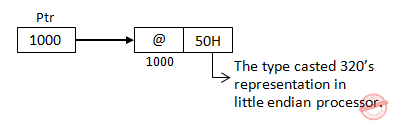Printf (“%d”, *ptr) ;
Output: 64 /* @ ASCII value is 64 */
Note: Assume short a is 2 byte integer.
 Question 14
Consider the following segment of C-code:The number of comparisons made in the execution of the loop for any n > 0 is:
 A ⌊log 2n⌋*n B n C ⌊log 2n⌋ D ⌊log 2n⌋+1
Programming-for-Output-Problems       Control Flow       ISRO-2016       Video-Explanation
Question 14 Explanation:
Explanation:
Let us consider n=6, then
1<=6 (correct)
2<=6 (correct)
4<=6 (correct)
8<=6 (False)
4 comparisons required
Option A:
⌊log n⌋+1
⌊log 6⌋+1
3+1=4 (correct)
Option B:
n=6 (False)
Option C:
⌊log n⌋
⌊log 6⌋=3 (False)
Option D:
⌊log 2n⌋+1
⌊log 26⌋+1=2+1=3 (False)
 Question 15
Consider the following C function:
int f(int n)
{
static int i = 1;
if(n >= 5) return n;
n = n+i;
i++;
return f(n);
}
The value returned by f(1) is
 A 5 B 6 C 7 D 8
Programming-for-Output-Problems       Programming       ISRO CS 2008
Question 15 Explanation:
The variable “i” is static variable and the value of that variable will be retained between multiple function calls.
first line of f() is executed only once.
Depending upon the value “n” and “i”, the function f() will until n>=5.
You can find the tracing of function f() from the below Execution of f(1)
i = 1
n = 2
i = 2
Call f(2)
i = 2
n = 4
i = 3
Call f(4)
i = 3
n = 7
i = 4
Call f(7)
since n >= 5 return n(7) with return value = 7
 Question 16
Consider the following code segment
for (int k=0; k<20; k=k+2)
{
if (k % 3 == 1)
system.out.print(k+ " ")
}
What is printed as a result of executing the code segment?
 A 4 16 B 4 10 16 C 0 6 12 18 D 1 4 7 10 13 16 19
Programming-for-Output-Problems       Programming       ISRO CS 2008
Question 16 Explanation:
for loop starts from 0 to 20 and increments with 2 at each iteration.
k = 0 % 3 = 0
k = 2 % 3 = 2
k = 4 % 3 = 1 // prints 4
k = 6 % 3 = 0
k = 8 % 3 = 2
k = 10 % 3 = 1 // prints 10
k = 12 % 3 = 0
k = 14 % 3 = 2
k = 16 % 3 = 1 // prints 16
k = 18 % 3 = 0
So, Output is 4 10 16
 Question 17
What is the output of this C++ program?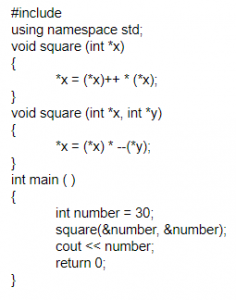A 910 B 920 C 870 D 900
Programming-for-Output-Problems       Functions       ISRO-2017 May       Video-Explanation
Question 17 Explanation:
Step-1: In main function, number variable has been initialized to 30.
Step-2: We pass address of number as parameters in the square function. As per the concept of function overloading in C++ the second square function will be executed.
Step-3: --(*y) will be executed first since decrement operator has the higher precedence than multiplication.
*x = (*x) * --(*y);
*x = 30 * 29;
*x = 870
Note: x is a pointer variable and it holds the address of the variable number.
 Question 18
The output of the following code is:
int main()
{
static int x[ ]={1,2,3,4,5,6,7,8}
int i;
for(i=2;i<6;++i)
x[x[i]]=x[i];
for(i=0;i<8;++i)
printf(“%d”,x[i]);
Return 0;
}
 A 12244668 B 11335578 C 12335578 D 12343676
Programming-for-Output-Problems       Nielit STA [02-12-2018]
Question 18 Explanation:Question 19
What will be the output of following?
main()
{
static int a=3;
printf("%d",a--);
if(a)
main();
}
 A 3 B 3 2 1 C 3 3 3 D Program will fall in continuous loop and print 3
Programming-for-Output-Problems       Storage-Classes       Nielit Scientific Assistance CS 15-10-2017
Question 19 Explanation:
Step-1: initial a=3 but we are given storage class is static.
Step-2: In printf( ) we are given post decrement. So, it will print 3 then decrements.
Step-3: if(2) then we are calling main() function, a=2 because of static otherwise it will be 3 only.
Step-4: It will print 2 and next iteration will print 1.
 Question 20
Consider the following code fragment
void foo(int x, int y)
{
x+=y;
y+=x;
}
main()
{
int x=5.5;
foo(x,x);
}
What is the final value of x in both call by value and call by reference, respectively?
 A 5 and 16 B 5 and 12 C 5 and 20 D 12 and 20
Programming-for-Output-Problems       Pointers       ISRO CS 2015       Video-Explanation
Question 20 Explanation:
Call by Value:
The call by value method of passing arguments to a function copies the actual value of an argument into the formal parameter of the function. In this case, changes made to the parameter inside the function have no effect on the argument.
foo(x,x) ---->foo(5,5)
Modification of x and y values won’t effect in the main program, So still x will hold value “5” because the data type is integer.
Call by Reference:
The call by reference method of passing arguments to a function copies the address of an argument into the formal parameter. Inside the function, the address is used to access the actual argument used in the call. It means the changes made to the parameter affect the passed argument.
Function call foo(x,x)---foo(5,5) calls the function
void foo(int x, int y)
{
x+=y;
y+=x;
}
Here x and y variables, both pointing to same variable “x” in the main function.
So, if any modification done in the function it automatically reflect in the main function.
x+=y means x=x+y=5+5=10 // The variable “x” of main function will hold value 10 which used by both local variables of x and y
y+=x means u=y+x= 10+10=20 // Y value is 10 because , it point to variable ”x” in the main function.
 Question 21
Consider the following C declaration
struct
{
short s;
union
{
float y;
long z;
}u;
}t;
Assume that the objects of the type short, float and long occupy 2 bytes, 4 bytes and 8 bytes, respectively. The memory requirement for variable t, ignoring alignment consideration, is
 A 22 bytes B 18 bytes C 14 bytes D 10 bytes
Programming-for-Output-Problems       Structure       ISRO CS 2015       Video-Explanation
Question 21 Explanation:
short  = 5×2 = 10 max[float, long] = max [4, 8] = 8 Total = short + max[float,long] = 10+8 = 18
 Question 22
Consider the following program fragment
if(a > b)
if(b > c)
s1;
else
s2;
s2 will be executed if
 A a <= b B b > c C b >= c and a <= b D a > b and b <= c
Programming-for-Output-Problems       if-else       ISRO CS 2015       Video-Explanation
Question 22 Explanation:
Here, possibility to happen “dangling if” error. But according to else statement it is matched with above if statement only.
We can write code segment is like this because to avoid “dangling if”
if(a>b)
{
if(b>c)
{ s1; }
else
{ s2; }
}
→ To execute s2, it (a>b) should be TRUE. And it (b>c) should be FALSE.
Hence, Option-D is correct
 Question 23
If n has 3, then the statement a[++n]=n++;
 A assigns 3 to a B assigns 4 to a C assigns 4 to a D what is assigned is compiler dependent
Programming-for-Output-Problems       Operator       ISRO CS 2015       Video-Explanation
Question 23 Explanation:
Given statement consists of three operators which are pre-increment , post-increment and equal to operator. According to operator precedence and associativity, the order of operators evaluation is post-increment,pre-increment and finally equal to operator. But one variable value is modifying multiple times in the same expression means that behaviour is undefined behaviour which is compiler dependent.
 Question 24
The following program
main()
{
inc();
inc();
inc();
}
inc()
{
static int x;
printf("%d", ++x);
}
 A prints 012 B prints 123 C prints 3 consecutive, but unpredictable numbers D prints 111
Programming-for-Output-Problems       Functions       ISRO CS 2015       Video-Explanation
Question 24 Explanation:
In this question, we have to remind one point about “static” storage class.
Static storage class by default value is 0.
Step-1: We are calling inc( ) in main function. So, it will enter into inc() function.
Here, we are using pre increment function, so it replaced 0 with 1.
Step-2: Second inc() in main function. So, it will enter into inc() function.
Here, we are using pre increment function, so it replaced 1 with 2.
Step-3: Third inc() in main function. So, it will enter into inc() function.
Here, we are using pre increment function, so it replaced 2 with 3.
Note: The static storage class instructs the compiler to keep a local variable in existence during the life-time of the program instead of creating and destroying it each time it comes into and goes out of scope.
 Question 25
Consider the following program fragment
i=6720;
j=4;
while (i%j==0)
{
i=i/j;
j=j+1;
}
On termination j will have the value
 A 4 B 8 C 9 D 6720
Programming-for-Output-Problems       Control Flow       ISRO CS 2015       Video-Explanation
Question 25 Explanation:
Initial values of i and j are 6720 and 4 respectively
Iteration 1:
i=6720
j=4
i%j==0 which is 6720%4 ==0 condition is true then
i=i/j=6720/4=1680
j=j+1=5
Iteration-2:
i=1630
j=5
i%j==0 which is 1680%5 ==0 condition is true then
i=i/j=1630/5=336
j=j+1=6
Iteration-3: i=336
j=6
i%j==0 which is 336%6 ==0 condition is true then
i=i/j=336/6=56
j=j+1=7
Iteration-4:
i=56
j=7
i%j==0 which is 56%7 ==0 condition is true then
i=i/j=56/7=8
j=j+1=8
Iteration-5:
i=8
j=8
i%j==0 which is 8%8 ==0 condition is true then
i=i/j=8/8=1
j=j+1=9
Iteration-6:
i=1
j=9
i%j==0 which is 1%9 ==0 condition is false.
At this iteration, the “j” value is 9.
 Question 26
Consider the following declaration: int a, *b=&a, **c=&b; The following program fragment a=4; **c=5;
 A does not change the value of a B assigns address of c to a C assigns the value of b to a D assigns 5 to a
Programming-for-Output-Problems       Pointers       ISRO CS 2015       Video-Explanation
Question 26 Explanation:
In the given program fragment, three variables are there
Ordinary variable -a // value will be stored
Pointer variable -b // address of variable will be stored

Pointer to pointer variable(double pointer) -c // address of pointer variable will stored.
a=4 means storing/assigning value to “a”.
**c means value at(value at(c)) =value at(value at(&b)) (c holds address of pointer “b”)
=value at(&a) (b holds the address of “a”)
Memory location of variable “a”, value 5 is stored/assigned into the variable.
 Question 27
The output of the following program is main() { static int x[] = {1,2,3,4,5,6,7,8} int i; for (i=2; i<6; ++i) x[x[i]]=x[i]; for (i=0; i<8; ++i) printf("%d", x[i]); }
 A 1 2 3 3 5 5 7 8 B 1 2 3 4 5 6 7 8 C 8 7 6 5 4 3 2 1 D 1 2 3 5 4 6 7 8
Programming-for-Output-Problems       Arrays       ISRO CS 2015       Video-Explanation
Question 27 Explanation:For loop will execute for the i value 2,3,4,5
For i = 2, x[x] = x
= x = 3 // since x = 3
The array elements representation areQuestion 28
Which of the following has the compilation error in C?
 A int n=17; B char c=99; C float f=(float)99.32; D #include
Programming-for-Output-Problems       Compilation       ISRO CS 2015       Video-Explanation
Question 28 Explanation:
Option A: General declaration of integer variable.
Option B: General declaration of character variable.
Option C: It is type casting. But given cast also same type.
Option D: We have to include a file otherwise it treated as compiler error.
 Question 29
The for loop for (i=0; i<10; ++i) printf("%d", i&1); prints:
 A 0101010101 B 0111111111 C 0000000000 D 1111111111
Programming-for-Output-Problems       Control Flow       ISRO CS 2015       Video-Explanation
Question 29 Explanation:
The loop will execute for 10 times, from “i” value 0 to 9
“&” is bitwise AND operator.For a given i=0 , the value is 0&1 is 0000 & 0001 which is 0
i=1, 0001 & 0001 which is 1
i=2 , 0010 & 0001 which is 0
i=3 0011 & 0001 which is 1
i=4 , 0100 & 0001 which is 0
i=5 0101 & 0001 which is 1
i=6 , 1100 & 0001 which is 0
i=7 0111 & 0001 which is 1
i=8 , 1000 & 0001 which is 0
i=9 1001 & 0001 which is 1
So the output is 0101010101(from i=0 to 9)
 Question 30
Consider the following C code segment:For the program fragment above, which of the following statements about the variables i and j must be true after the execution of this program? [!(exclamation) sign denotes factorial in the Solution]
 A ( j=(x-1)!) ∧ (i>=x) B ( j = 9!) ∧ (i =10) C (( j = 10!) ∧ (i = 10 )) V (( j = (x - 1)!) ∧ (i = x )) D (( j = 9!) ∧ (i = 10)) V (( j = (x - 1)!) ∧ (i = x ))
Programming-for-Output-Problems       Control Flow       ISRO-2018       Video-Explanation
Question 30 Explanation:
As per code segment,
Step-1: The ith loop will compute from value 1 to 9. If the condition is true, then it computes j=j*i statement.
Step-2: The statement j=j*i is nothing but calculating 9!. If the condition fails automatically while loop will be terminated. It means maximum we can execute 9 values.
Step-3: when x becomes 10 then maximum I value will be 9. So, we can calculate up to 9! And I become 10 then it terminates the condition.
 Question 31
Assume A and B are non zero positive integers. The following code segment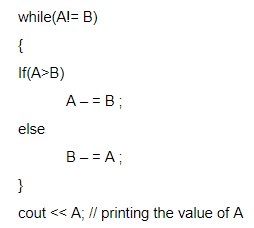A Computes the LCM of two numbers B Divides the larger number by the smaller number C Computes the GCD of two numbers D Finds the smaller of two numbers
Programming-for-Output-Problems       Control Flow       ISRO-2018       Video-Explanation
Question 31 Explanation:
→ The above iterative code is computes the GCD of two numbers using euclidean algorithm.
→ The procedure is to subtract smaller number from larger. So that we can reduce larger number then doesn’t change the value of GCD.
→ if we performing number of iterations based on condition the larger of two numbers will end up with GCD.
 Question 32
What is the output of the following program ?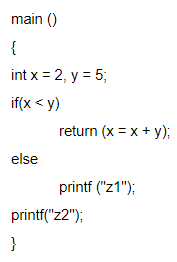A z2 B z1z2 C Compilation error D None of these
Programming-for-Output-Problems       if-else       ISRO-2018       Video-Explanation
Question 32 Explanation: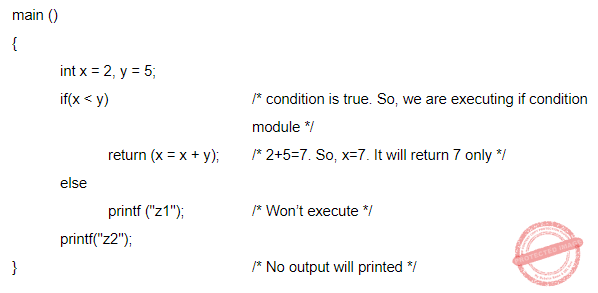Question 33
Consider the following programAssuming required header files are included and if the machine in which this program is executed is little-endian, then the output will be
 A 0 B 99999999 C 1 D unpredictable
Programming-for-Output-Problems       Pointers       ISRO-2018       Video-Explanation
Question 33 Explanation: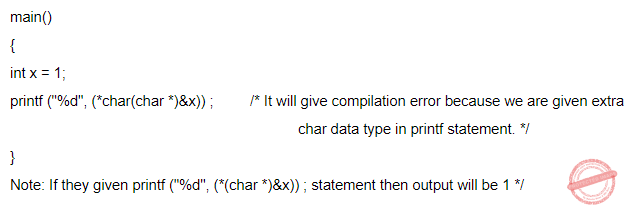Question 34
Consider the following declaration:A person.name +2 B kd → (name +2 ) C *((*kd).name + 2 ) D either (a) or (b), but not (c)
Programming-for-Output-Problems       Structure       ISRO-2018       Video-Explanation
Question 34 Explanation:
*(kd -> name +2) /* this is wrong statement */
We have to write *((*kd).name+2).
Note: They are specifically asked about a value stored at that location of the string by using "*" outside the brackets. Otherwise, option A and B would be correct.
 Question 35
Consider the following C programHow many lines of output does this program produce?
 A 0-9 lines of output B 10-19 lines of output C 20-29 lines of output D More than 29 lines of output
Programming-for-Output-Problems       Control Flow       ISRO-2018       Video-Explanation
Question 35 Explanation:
→ Since there is one line of output for each loop, we need to determine the number of
times the loop executes. Since i is constant, we need to see the growth of j only.
→ Let the initial value of j be denoted by J1 and the subsequent values by Jn for n= 2, 3,... so that J denotes a progression of j. We see that Jn = 2Jn-1;
→ J1 = 2, which gives Jn = 2n-1;
n = 1, 2, ...
→ The loop will execute as long as i/j=2.0/2n-1 >0.001 which gives n<3log210+2 (or) n<11.97.
→ Thus the loop will execute 11 times which is equivalent to say there will be 11 lines of output.
There are 35 questions to complete.

Register Now